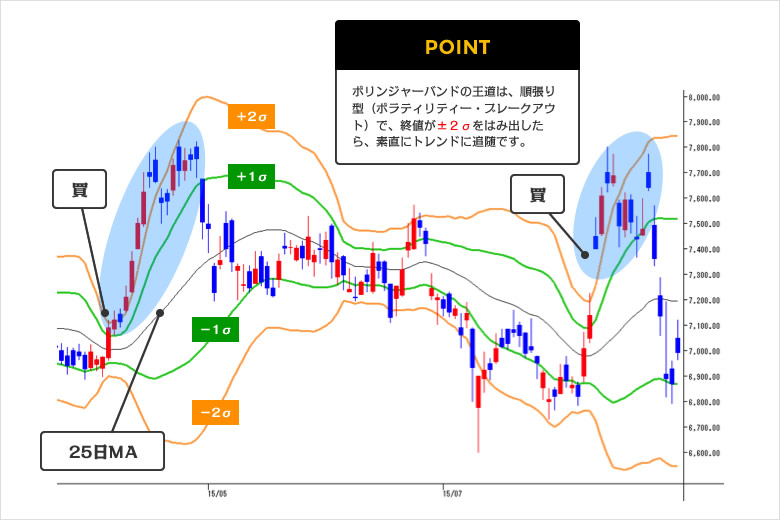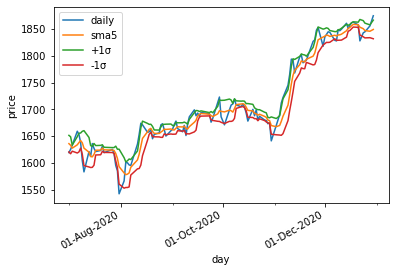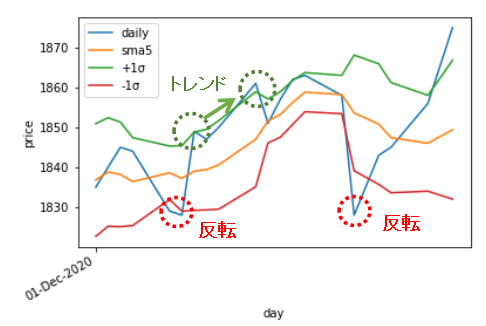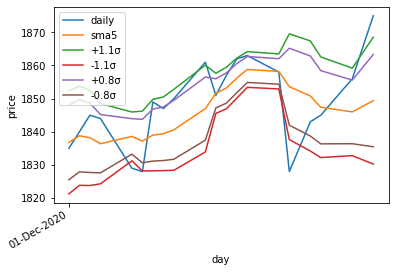# [PYTHON] Investment quest: Make a system trade with pyhton (2)

## System trading in python: Draw Bollinger Bands

### What is Bollinger Bands?

Bollinger Bands are often described in the graph below. From monex Securities HP. https://info.monex.co.jp/technical-analysis/indicators/003.htmlI often see pictures on the website of a securities company, but I have a question here. why? 25 moving averages (25SMA), why ± 1, 2σ? There may be an optimum value for odd values ​​such as 5SMA, 7SMA, 0.9σ, 1.4σ, and so on. Since this is the world of DX, it is easy to calculate with the power of your home computer. I came up with the idea last night to try to find the optimum values ​​for this σ and SMA. I don't know if it will work just by thinking about it ... To do that, you first need to draw a Bollinger band.

### Draw Bollinger Bands

To draw the Bollinger Bands, use `rolling` to find the standard deviation, just as you would draw a moving average. `df["5day_std"]=df["end_price"].rolling(5).std().round(1)` Simply add or subtract this standard deviation from the mean. If + 1σ, `df["5day_bol_+1"]=df["5day_ave"]+df["5day_std"]` is.

``````#Find the standard deviation
df["5day_std"]=df["end_price"].rolling(5).std().round(1)
#Find the Bollinger Bands (± 1σ)
df["5day_bol_+1"]=df["5day_ave"]+df["5day_std"]
df["5day_bol_-1"]=df["5day_ave"]-df["5day_std"]

``````

Now, let's draw a graph for the second half of FY2008.I will take a closer look. This is the situation in December 2008. There are places where it is rising and places where it is reversing following the trend.This graph is ± 1σ, but since it is a computer, you can calculate as much as you like. For example, ± 0.8σ or ± 1.1σ.I would like to find the optimum value by the number of days and bandwidth of this moving average (SMA).

The first simple strategy is

Make a short-term game. The number of days on the moving average is several days. Search for the optimal solution for bandwidth and number of days to invert or trend in Bollinger Bands.